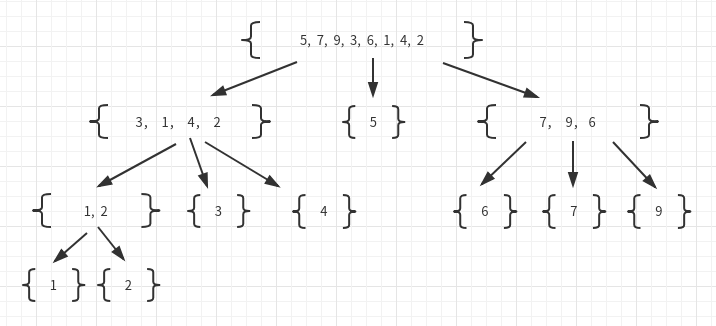# 快速排序python实现

## 快速排序## 具体算法


def quick_sort(s):
"""快速排序，s为列表"""
# 结束条件
if len(s) < 2:
return
# 从列表取出一个元素作为基准值
p = s
L = [] # 小于
E = [] # 等于
R = [] # 大于
# 把s里的元素放入3个队列
while len(s) > 0:
if s[-1] < p:
L.append(s.pop())
elif s[-1] == p:
E.append(s.pop())
else:
R.append(s.pop())

quick_sort(L)
quick_sort(R)
s.extend(L)
s.extend(E)
s.extend(R)

if __name__ == '__main__':
s = [1, 7, 3, 5, 4]
quick_sort(s)
print(s)


## 就地快速排序


def inplace_quick_sort(s,a,b):
"""列表的就地快速排序，s为列表，a为起始索引，b为终止索引"""
if a >= b:
return
# s[b]作为基准值
p = s[b]
# left和right相当于指向
left = a
right = b-1
# 把除了s[b]d 其他元素按照以s[b]为基准分割
while left <= right:

while left <= right and s[left] < p:
left += 1

while left <= right and p < s[right]:
right -=1

if left <= right:
s[left],s[right] = s[right],s[left]
left,right = left+1,right-1

s[left],s[b] = s[b],s[left]
inplace_quick_sort(s,a,left-1)
inplace_quick_sort(s,left+1,b)


• 选择索引b处的值为基准值

• 通过从左到右扫描与从右到左扫描，left是左扫描位置，right是右扫描位置，找到左边第一个大于基准值的位置与右边第一个小于基准值的位置

• 然后将这两个值交换，并将left向右移动，right向左移动，继续重复进行

• 直到left>right,也就是全部扫描一遍后，将基准值s[b]与最后的left位置交换

• 这样就完成了分割

• 然后再进行递归调用两个序列

posted @ 2019-03-26 18:43  sfencs  阅读(2838)  评论(0编辑  收藏